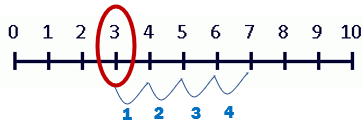# Math Worksheets Land

Math Worksheets For All Ages

# Math Worksheets Land

Math Worksheets For All Ages

Home > Math Topics > Addition >

# Adding on a Number Line Worksheets

A number line is a great tool for introducing addition to students. I would highly suggest using standardized classroom version that your students can become comfortable with. I would suggest starting with a single intervals which starts at 0 and ends at 10. You can move to mixed intervals as your students have more success with this. Students will then learn to pick one of the addends as the starting point on the number line. The second step is to count up the number indicated by the other addend. The integer that you arrive at is the value of the sum of these two integers. I find that using this tool really helps students grasp the concept of addition. I do find them to not help long term memory of sums, that is better served by addition tables and math facts flashcards. This series of worksheets has students model addition with the use of a numbers line.

### Aligned Standard: K.NBT.A.1

• Mixed Addition Step-by-Step Lesson- Numbers lines always come in handy for addition and subtraction too.
• Guided Lesson - We have you make and read a number line to solve a series of sums.
• Guided Lesson Explanation - You need some space to do these problems. See how this works out for you.
• Practice Sheet - The problems are set in a nice bold font that is easy to work with.
• Matching Worksheet - This one was tough to put together. It was a request from a teacher in Florida. I played around with just providing one line, but it wasn't as effective.

There is a plethora of tools that help in simplifying mathematical problems and the concept that appear over the course of a year in school. These tools can make it easy for students to solve problems in a shorter amount of time without the need for extra effort. One such tool is a number line. It is a tool that is easy to use and can make it easy for students to learn to add, subtract, and even multiply numbers.

A number line is a series of numbers that are placed on a straight line in an ascending order into a selection of fixed sections of equal intervals. Students can make a number line as per the question they are solving. A number line can be used to represent both positive as well as negative integers. You can use a number line for a great deal more than just creating a set interval.

When adding two numbers using a number line, what a student must do is locate the first number on the number line and then use a pencil to make skips equal to the second number which is the addend of the addition problem. This indicates the movement of a single interval. Addition can be seen as an exercise in counting where you identify one of the addends and then count up the number identified by the other addend to determine the sum of the two addends.

For example, of you are adding 3 + 4. You would need to use a number line that has at least 10 intervals to give yourself some workspace. You would locate 3 as the starting position on the number line and make 4 skips to get the final answer (7). Take look at how this unfolds on the number line below.Unlock all the answers, worksheets, homework, tests and more!
Save Tons of Time! Make My Life Easier Now

## Thanks and Don't Forget To Tell Your Friends!

I would appreciate everyone letting me know if you find any errors. I'm getting a little older these days and my eyes are going. Please contact me, to let me know. I'll fix it ASAP.